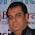### vlookup formula in excel, vlookup formula logic with example

vlookup is a formula in excel, commonly used to retrieve data form one table to another table.

In this article, we will provide complete information about vlookup formula.

# Why vlookup in excel? vlookup function

Suppose you are in a condition, where you need some information on table “A” from table “B”

For example, in below tables, Table A have column “Name”, “Age”& “Salary” and table B have “Name”, “Age”& “Project”.

So to copy "project" column of employees given in “Table B”, we can use vlooup formula or vlookup function.

## vlookup formula Logic

We need to first understand the logic of vlookup function then we can jump to calculation part.

Vlookup function have four parameters, it is very important to understand these parameters before using it.

1. lookup_value
2. table_array
3. col_index_num
4. Range_lookup

### lookup_value :

It defines the identifier cell number. Identifier cell number is a common column present in both table and it is used to compare the tables.

To start with we lookup, first we need to identify “primary key”/”identifier” column which is present in both tables.

Here our identifier is column “Name” because it is present in both table(This is termed as lookup_value in formula)

As we have already identified Column “Name” as identifier hence cell “A3” from “Table A” will be a lookup value.

### table_array  :

This parameter defines range of the table from where data needs to be copied.

Here we need to select entire "table B" in order to get results.

Note: Selection only needs to be started from “identifier column” to result column.

### col_index_number :

This parameter defines position of column from where you need to retrieve data. In below example, column "project" is in 3rd position hence the value will be”3″.

### range_lookup :

Range lookup defines if matching should be exact or Approx.

You can write “True/False” or “0/1” to get result where 0 defines “Exact match” and “1” defines “approx match”.

We will use “Exact match” here for accurate result. (Recommended)

Now we are ready with in vlookup function  "=VLOOKUP(A3,J3:L7,1,0)"

Note: all parameters needs to be separated by “,”

Now can now simply drag this formula to other rows and then you are ready with your data.

#### 5 comments:

1.This content is really unique and amazing. This article really helpful and explained very well.

Learn MIS Training Core to Advanced Level

2.Excellent Blog! I would like to thank for the efforts you have made in writing this post. I am hoping the same best work from you in the future as well.

Get Learn AutoCAD Training with 20% Off

3.Excellent post. I was always checking this blog, and I’m impressed! Extremely useful info specially the last part, I care for such information a lot about Addon domain.

Best Training Institute for SQL Server Training

4.A really appreciable content you put in your blog and detailed information you provide helps me to enhance my knowledge and skills. Further More Information About Advance Excel Training Institute in Delhi So Contact Here-+91-9311002620 Or Visit Website- https://htsindia.com/Courses/business-analytics/adv-excel-training-course.

5.Thanks for posting these kinds of post its very helpful and very good content a really appreciable post apart from that if anyone looking for best Core and Advanced Java training institute in delhi so contact here +91-9311002620 visit https://www.htsindia.com/java-training-courses